### Stress: Stress Measures and Stress Invariants

#### Learning Outcomes

• Differentiate between the matrix representation of stress and the various measures of stress at a point.
• Calculate the Hydrostatic Stress, the deviatoric stress tensor, and the von Mises stress, given a stress matrix.
• Compute the value and the orientation of the maximum shear stress for a 3d state of stress.

The following the basic measures of stress along with the invariants of the stress matrix:

### Hydrostatic Stress

The hydrostatic stress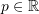at a point is a real number representing the average of the normal stresses on the faces of an infinitesimal cube. This average is independent of the coordinate system used since it is equal to one third of the trace (or the first invariant) of the stress tensor. If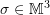is a stress matrix and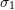,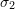and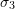are the principal stresses, then the hydrostatic stress is equal to:

(1)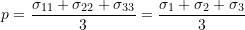### Deviatoric Stress Tensor

The deviatoric stress tensor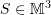is a measure of stress which, in linear elastic materials, is considered to be responsible for changing the shape of the material while keeping the volume constant. In other words, it is responsible for shearing the material. The deviatoric stress tensor is defined as:

(2)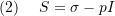where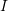is the identity tensor.

### Von Mises Stress

The von Mises stress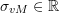is a real number and a function of the second invariant of the deviatoric stress tensor, namely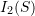. It plays a major role in defining the onset of failure or yield of traditional linear elastic materials. It is defined as: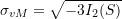Recall that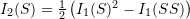. From (1) and (2) above,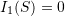, then,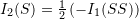. Thus,

(3)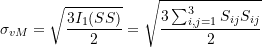Notice that since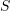is symmetric, the following equality was utilized: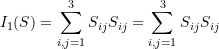Using (1) and (2) to replace the components ofwith components ofin the expression for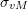results in the following equivalent expression for: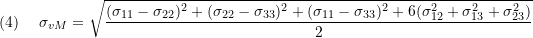In the principal coordinate system described by the eigenvectors of, the following equivalent expression foris more convenient to use: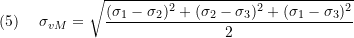### Maximum Shear Stress

As shown above, the function of the stress matrix is to obtain the force per unit area acting on a particular plane at the point of interest. As the orientation of the plane changes, the shear stress and the normal stress on that plane change as well. The maximum shear stress is a real number that represents the maximum shear stress that will exist after reorientation of the plane. It is equal to half the maximum difference between the principal stresses: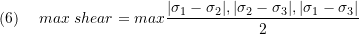The maximum shear stress is attained on a plane inclined by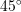to the two principal planes (defined by the principal stresses directions) associated with the maximum difference in the principal stresses. This can be shown by considering a plane problem aligned with the principal directions. the stress matrix in this case is given by: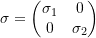A general counterclockwise coordinate transformation matrix has the form: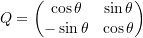The stress matrix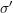after coordinate transformation has the form: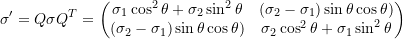The maximum value for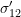is obtained by finding the angle that would satisfy: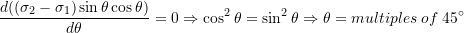In that case, the value of the shear stress component: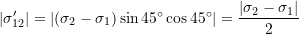The following Mathematica code shows the above calculations:

View Mathematica Code

Clear[s1,ss,s2, theta]
ss={{s1,0},{0,s2}};
Q=RotationMatrix[-theta];
news=FullSimplify[Q.ss.Transpose[Q]];
Q//MatrixForm
news//MatrixForm
eq1=D[news[[1,2]],theta];
Solve[eq1==0,theta]

View Python Code

from sympy import Matrix,simplify,diff,solve
import sympy as sp
s1,s2,t = sp.symbols("sigma_1 sigma_2 theta")
ss = Matrix([[s1,0],[0,s2]])
display("\u03C3 =",ss)
Q = Matrix([[sp.cos(t), -sp.sin(t)],
[sp.sin(t), sp.cos(t)]])
display("Q =",Q)
news = simplify(Q*ss*Q.T)
display("Stress Matrix")
display("\u03C3' =",news)
eq1 = diff(news[0,1],t)
display("Max value of \u03C3'_12 =",eq1)
s = solve(eq1,t)
display("\u03B8 =",s,"degrees: ",[i*180/sp.pi for i in s])


In the following two dimensional example, a square is viewed in the coordinate system described by the principal stresses directions. In this coordinate system, the forces acting on the square faces are perpendicular to those faces (i.e., no shear stresses exist on those faces). If the square is aligned with another coordinate system aligned atwith the principal coordinate system, the maximum shear stress is attained. Change the values of the stress matrix entries and press enter to see how the results are affected. Find a combination of the stress matrix entries that are not all equal to zero such that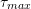is equal to zero.

In the figure below, the red and blue arrows indicate the traction vectors acting on the planes perpendicular to the first and second basis vectors respectively. The original coordinate system is defined by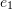and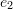. The principal coordinate system is defined by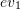and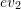while the coordinate system in which the perpendicular planes have maximum shear is defined by the two vectors ems1 and ems2.

The following is a three dimensional example. Test your knowledge by changing the numerical values and observing the orientation of the principal planes and the planes with maximum shear stress.

Note that the principal stresses (eigenvalues) and their corresponding principal directions (eigenvectors) are ordered by increasingly sorting the principal stresses, i.e.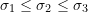. Therefore, the maximum shear is always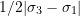, and the maximum shear plane is then attained by 45 degrees of rotation of the (first and third) principal directions about the axis of.

### Numerical Example

Change the entries for the components of the stress matrix and evaluate the different stress measures and invariants defined above: